Courses

# Taylor Series Mathematics Notes | EduRev

## Mathematics : Taylor Series Mathematics Notes | EduRev

The document Taylor Series Mathematics Notes | EduRev is a part of the Mathematics Course Algebra for IIT JAM Mathematics.
All you need of Mathematics at this link: Mathematics

In the previous section we started looking at writing down a power series representation of a function. The problem with the approach in that section is that everything came down to needing to be able to relate the function in some way to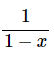and while there are many functions out there that can be related to this function there are many more that simply can’t be related to this.
So, without taking anything away from the process we looked at in the previous section, what we need to do is come up with a more general method for writing a power series representation for a function.
So, for the time being, let’s make two assumptions. First, let’s assume that the function f(x) does in fact have a power series representation about x=a,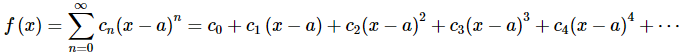Next, we will need to assume that the function, f(x), has derivatives of every order and that we can in fact find them all.
Now that we’ve assumed that a power series representation exists we need to determine what the coefficients, cn, are. This is easier than it might at first appear to be. Let’s first just evaluate everything at x=a. This gives,
f(a)=c0
So, all the terms except the first are zero and we now know what c0 is. Unfortunately, there isn’t any other value of x that we can plug into the function that will allow us to quickly find any of the other coefficients. However, if we take the derivative of the function (and its power series) then plug in x=a we get,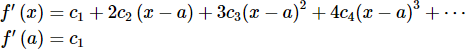and we now know c1.
Let’s continue with this idea and find the second derivative.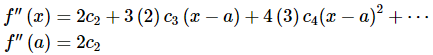So, it looks like,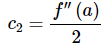Using the third derivative gives,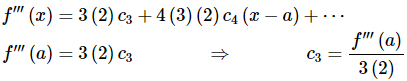Using the fourth derivative gives,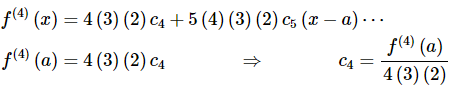Hopefully by this time you’ve seen the pattern here. It looks like, in general, we’ve got the following formula for the coefficients.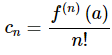This even works for n=0 if you recall that 0!=1 and define f(0)(x)=f(x).
So, provided a power series representation for the function f(x) about x=a exists the Taylor Series for f(x) about x=a is,
Taylor Series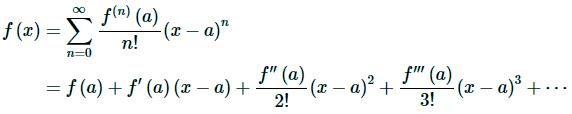If we use a=0, so we are talking about the Taylor Series about x=0, we call the series a Maclaurin Series for f(x) or,
Maclaurin Series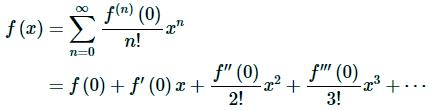Before working any examples of Taylor Series we first need to address the assumption that a Taylor Series will in fact exist for a given function. Let’s start out with some notation and definitions that we’ll need.
To determine a condition that must be true in order for a Taylor series to exist for a function let’s first define the nth degree Taylor polynomial of f(x) as,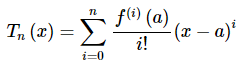Note that this really is a polynomial of degree at most n. If we were to write out the sum without the summation notation this would clearly be an nth degree polynomial. We’ll see a nice application of Taylor polynomials in the next section.
Notice as well that for the full Taylor Series,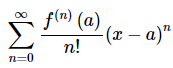the nth degree Taylor polynomial is just the partial sum for the series.
Next, the remainder is defined to be,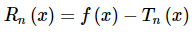So, the remainder is really just the error between the function f(x) and the nth degree Taylor polynomial for a given n.
With this definition note that we can then write the function as,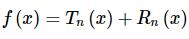We now have the following Theorem.
Theorem
Suppose that f(x)=Tn(x)+Rn(x). Then if,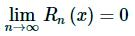for |x−a|<R then,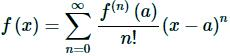on |x−a|<R.
In general, showing that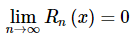is a somewhat difficult process and so we will be assuming that this can be done for some R in all of the examples that we’ll be looking at.
Now let’s look at some examples.

Example 1: Find the Taylor Series for f(x)=ex about x=0.
Solution. This is actually one of the easier Taylor Series that we’ll be asked to compute. To find the Taylor Series for a function we will need to determine a general formula for f(n)(a). This is one of the few functions where this is easy to do right from the start.
To get a formula for f(n)(0) all we need to do is recognize that,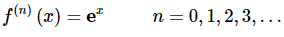and so,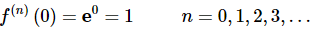Therefore, the Taylor series for f(x)=ex about x=0 is,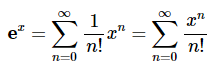Example 2: Find the Taylor Series for f(x)=e−x about x=0.
There are two ways to do this problem. Both are fairly simple, however one of them requires significantly less work. We’ll work both solutions since the longer one has some nice ideas that we’ll see in other examples.
Solution 1
As with the first example we’ll need to get a formula for f(n)(0). However, unlike the first one we’ve got a little more work to do. Let’s first take some derivatives and evaluate them at x=0.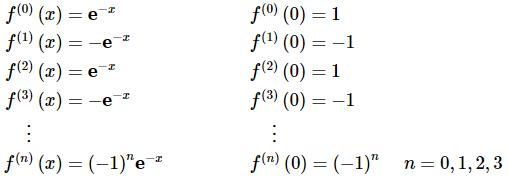After a couple of computations we were able to get general formulas for both
f(n) (x)
and
f(n) (0)
We often won’t be able to get a general formula for
f(n) (x)
so don’t get too excited about getting that formula. Also, as we will see it won’t always be easy to get a general formula for
f(n) (a)
So, in this case we’ve got general formulas so all we need to do is plug these into the Taylor Series formula and be done with the problem.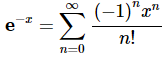Solution 2
The previous solution wasn’t too bad and we often have to do things in that manner. However, in this case there is a much shorter solution method. In the previous section we used series that we’ve already found to help us find a new series. Let’s do the same thing with this one. We already know a Taylor Series for ex about x=0 and in this case the only difference is we’ve got a “-x” in the exponent instead of just an x.
So, all we need to do is replace the x in the Taylor Series that we found in the first example with “-x”.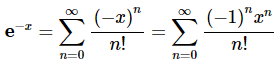This is a much shorter method of arriving at the same answer so don’t forget about using previously computed series where possible (and allowed of course).

Example 3: Find the Taylor Series for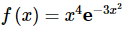about x=0.
Solution. For this example, we will take advantage of the fact that we already have a Taylor Series for ex about x=0. In this example, unlike the previous example, doing this directly would be significantly longer and more difficult.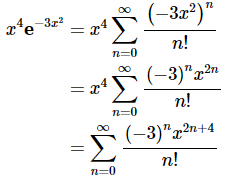To this point we’ve only looked at Taylor Series about x=0 (also known as Maclaurin Series) so let’s take a look at a Taylor Series that isn’t about x=0. Also, we’ll pick on the exponential function one more time since it makes some of the work easier. This will be the final Taylor Series for exponentials in this section.

Example 4: Find the Taylor Series for f(x) =e−x about x=−4.
Solution. Finding a general formula for f(n)(−4) is fairly simple.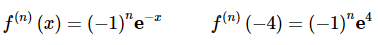The Taylor Series is then,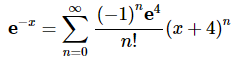Okay, we now need to work some examples that don’t involve the exponential function since these will tend to require a little more work.

Example 5: Find the Taylor Series for f(x)=cos(x) about x=0.
Solution. First, we’ll need to take some derivatives of the function and evaluate them at x=0.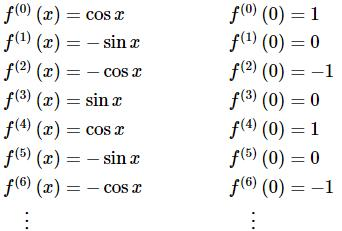In this example, unlike the previous ones, there is not an easy formula for either the general derivative or the evaluation of the derivative. However, there is a clear pattern to the evaluations. So, let’s plug what we’ve got into the Taylor series and see what we get,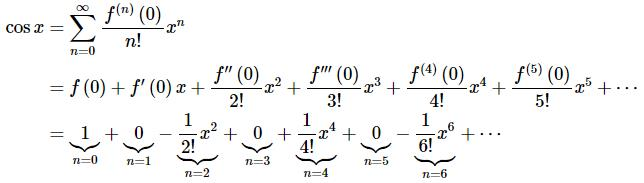So, we only pick up terms with even powers on the x’s. This doesn’t really help us to get a general formula for the Taylor Series. However, let’s drop the zeroes and “renumber” the terms as follows to see what we can get.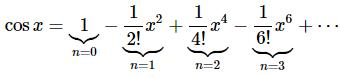By renumbering the terms as we did we can actually come up with a general formula for the Taylor Series and here it is,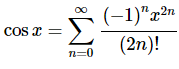This idea of renumbering the series terms as we did in the previous example isn’t used all that often, but occasionally is very useful. There is one more series where we need to do it so let’s take a look at that so we can get one more example down of renumbering series terms.

Example 6: Find the Taylor Series for f(x)=sin(x) about x=0.
Solution. As with the last example we’ll start off in the same manner.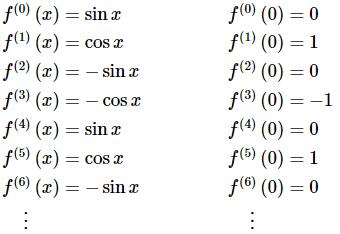So, we get a similar pattern for this one. Let’s plug the numbers into the Taylor Series.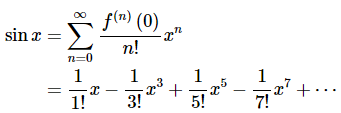In this case we only get terms that have an odd exponent on x and as with the last problem once we ignore the zero terms there is a clear pattern and formula. So renumbering the terms as we did in the previous example we get the following Taylor Series.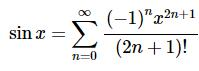We really need to work another example or two in which f(x) isn’t about x=0.

Example 7: Find the Taylor Series for f(x)=ln(x) about x=2.
Solution. Here are the first few derivatives and the evaluations.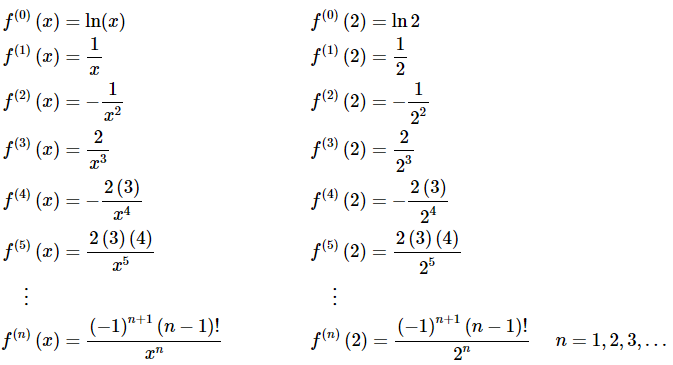Note that while we got a general formula here it doesn’t work for n=0. This will happen on occasion so don’t worry about it when it does.
In order to plug this into the Taylor Series formula we’ll need to strip out the n=0 term first.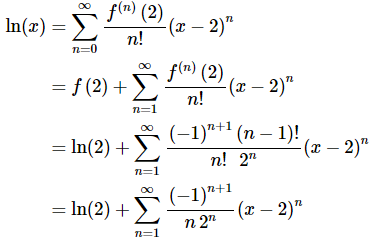Notice that we simplified the factorials in this case. You should always simplify them if there are more than one and it’s possible to simplify them.
Also, do not get excited about the term sitting in front of the series. Sometimes we need to do that when we can’t get a general formula that will hold for all values of n.

Example 8: Find the Taylor Series for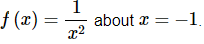Solution. Again, here are the derivatives and evaluations.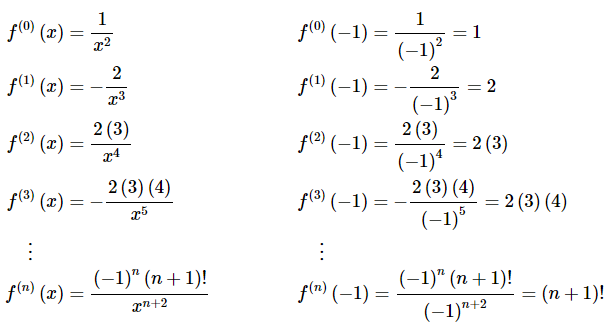Notice that all the negative signs will cancel out in the evaluation. Also, this formula will work for all n, unlike the previous example.
Here is the Taylor Series for this function.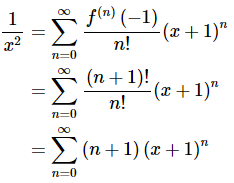Now, let’s work one of the easier examples in this section. The problem for most students is that it may not appear to be that easy (or maybe it will appear to be too easy) at first glance.

Example 9: Find the Taylor Series for f(x)=x3−10x2+6 about x=3.
Solution. Here are the derivatives for this problem.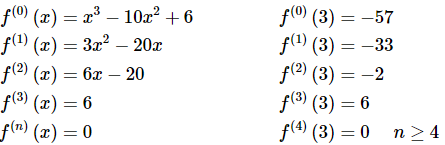This Taylor series will terminate after n=3. This will always happen when we are finding the Taylor Series of a polynomial. Here is the Taylor Series for this one.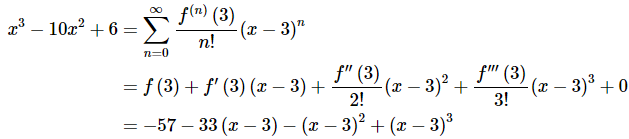When finding the Taylor Series of a polynomial we don’t do any simplification of the right-hand side. We leave it like it is. In fact, if we were to multiply everything out we just get back to the original polynomial!
While it’s not apparent that writing the Taylor Series for a polynomial is useful there are times where this needs to be done. The problem is that they are beyond the scope of this course and so aren’t covered here. For example, there is one application to series in the field of Differential Equations where this needs to be done on occasion.
So, we’ve seen quite a few examples of Taylor Series to this point and in all of them we were able to find general formulas for the series. This won’t always be the case. To see an example of one that doesn’t have a general formula check out the last example in the next section.
Before leaving this section there are three important Taylor Series that we’ve derived in this section that we should summarize up in one place. In my class I will assume that you know these formulas from this point on.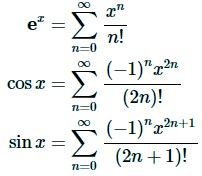Offer running on EduRev: Apply code STAYHOME200 to get INR 200 off on our premium plan EduRev Infinity!

58 docs

,

,

,

,

,

,

,

,

,

,

,

,

,

,

,

,

,

,

,

,

,

;